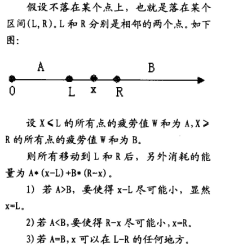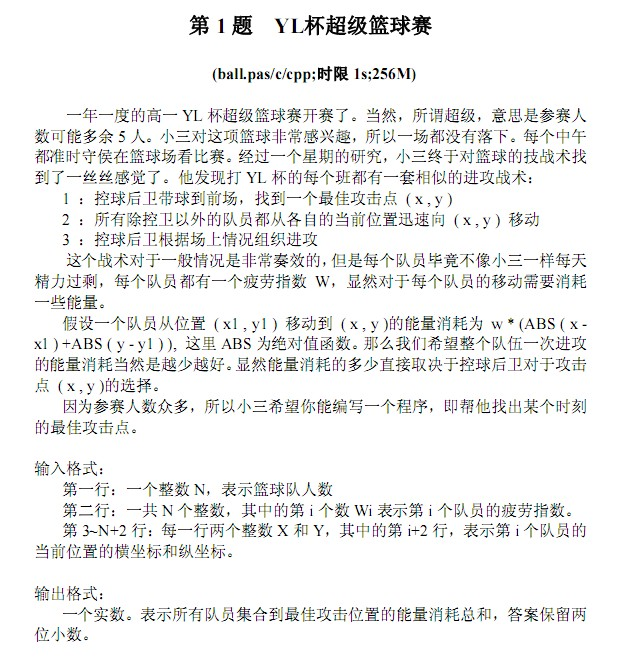• ## 关于区间中位数

千次阅读 2019-10-23 20:45:03
区间，求出他们的中位数。 题解 这个ider先在这里占坑吧，毕竟感觉这个思路挺好的。 我们可以一开始把整个序列排序，然后对排序后的数组链表化，并且求出每个点在链表中的下标。 那么对于固定的 l l l ，...
参考文献
洛谷某讨论https://www.luogu.org/discuss/show/158501?page=2
题意
对于一串长度为$n(n<=2000)$的序列，对于所有的$i,j(1<=i<=n,i<=j<=n)$区间，求出他们的中位数。
题解
这个ider先在这里占坑吧，毕竟感觉这个思路挺好的。
我们可以一开始把整个序列排序，然后对排序后的数组链表化，并且求出每个点在链表中的下标。
那么对于固定的$l$，我们的$r$从大到小查找，我们对于$[l,n]$暴力在链表找中位数，然后对于$[l,n-1]$我们可以把$n$在链表中删掉，然后看看中位数向左还是向右，这个是$O(1)$的。
然后我们可以$O(1)$将$[l,n]$的排序链表推到$[l+1,n]$的排序链表，不过我们需要拷贝数组，为$O(n)$，当然我朋友的代码是递归实现，所以不用拷贝。
代码来自HYY大神的，当然也是他最先提出这个问题。
注意：至于偶数的中位数，貌似这位神犇的代码是取左边的那个数字
#include<cstdio>
#include<algorithm>
using namespace std;
const int N=2005;
int a[N],p[N],l[N],r[N],w[N];
bool cmp(int x,int y){return a[x]<a[y];}
int f[N][N];
void dfs(int i,int k,bool bk,int now)
{
if(i<=k)
{
int j=w[k];//printf("%d\n",j);
if(bk){if(j<=now)now=r[now];}
else{if(j>=now)now=l[now];}
int r1=r[l[j]],l1=l[r[j]];//tmp
r[l[j]]=r[j];l[r[j]]=l[j];
f[i][k-1]=a[p[now]];
dfs(i,k-1,bk^1,now/*目前中位数的位置*/);
r[l[j]]=r1;l[r[j]]=l1;
}
}
void make(int n)
{
bool bk;
if(n&1)bk=false;//奇数为false
else bk=true;
int now=(n+1)/2;
f[n]=a[p[now]];dfs(1,n,bk,now);
for(int i=1;i<n;i++)
{
int j=w[i];//printf("%d\n",j);
if(bk){if(j<=now)now=r[now];}
else{if(j>=now)now=l[now];}
r[l[j]]=r[j];l[r[j]]=l[j];bk^=1/*奇偶性质*/;//删数
f[i+1][n]=a[p[now]];
dfs(i+1,n,bk,now);//处理中位数
}
}
int main()
{
int n;scanf("%d",&n);
for(int i=1;i<=n;i++)scanf("%d",&a[i]);
for(int i=1;i<=n;i++)p[i]=i;
sort(p+1,p+n+1,cmp);
for(int i=1;i<=n;i++)w[p[i]]=i;
for(int i=1;i<=n;i++)l[i]=i-1,r[i]=i+1;
make(n);
for(int i=1;i<=n;i++)
{
for(int j=i;j<n;j++)printf("%d ",f[i][j]);
printf("%d\n",f[i][n]);
}
return 0;
}



展开全文• 在算法竞赛，有一类求出给定区间符合要求的的个数问题，这类问题往往区间范围较大，无法通过枚举区间中数再判断条件这种方式来求解，数位dp就是一种解决这种方式的策略。 给出一篇写的很好地文章链接总体策略...

引言
总体策略
不要62
Bomb
B-number
Balanced Number

引言

在算法竞赛中，有一类求出给定区间中符合要求的数的个数问题，这类问题往往区间范围较大，无法通过枚举区间中数再判断条件这种方式来求解，数位dp就是一种解决这种方式的策略。

给出一篇写的很好地文章链接

总体策略

若区间符合可加减，
求解[l,r]<!--//--><![CDATA[//><!--
[l, r]
//--><!]]>满足条件的数个数可以通过[0,r]−[0,l−1]<!--//--><![CDATA[//><!--
[0, r] - [0, l-1]
//--><!]]>来完成

问题转化为求解[0,n]<!--//--><![CDATA[//><!--
[0, n]
//--><!]]>
对于一个小于n的数，必然从高位到低位有一位数小于n中对应位置上的数，这样我们在循环时可以通过枚举每一位数上的数字范围来求解。

不要62

题目链接

杭州人称那些傻乎乎粘嗒嗒的人为62（音：laoer）。
杭州交通管理局经常会扩充一些的士车牌照，新近出来一个好消息，以后上牌照，不再含有不吉利的数字了，这样一来，就可以消除个别的士司机和乘客的心理障碍，更安全地服务大众。
不吉利的数字为所有含有4或62的号码。例如： 62315 73418 88914
都属于不吉利号码。但是，61152虽然含有6和2，但不是62连号，所以不属于不吉利数字之列。
你的任务是，对于每次给出的一个牌照区间号，推断出交管局今次又要实际上给多少辆新的士车上牌照了。

思路：
定义dp[i][j]<!--//--><![CDATA[//><!--
dp[i][j]
//--><!]]>表示以j开头位数为i的满足条件的数的个数,
初始化dp =1, 接着预处理完成赋值，包含前导0。

    dp = 1;
for(i=1; i<=7; i++)     //循环多少位数
{
for(j=0; j<=9; j++)     //循环第i位数字取值
{
for(k=0; k<=9; k++)     //循环第i-1位数字取值
{
if(j!=4 && !(j==6 && k==2))
{
dp[i][j] += dp[i-1][k];
}
}
}
}

递推时由低位向高位枚举，逐步求出所有dp值

通过区间相减方式区间个数问题变为[0, x]间个数问题，统计数字前用v数组记录x每一位数字值。

for(i=size; i>0; i--)
{
for(j=v[i-1]-1; j>=0; j--)
{
if(j!=4 && !(j==2 && v[i] == 6))
{
ans += dp[i][j];
}
}

if(v[i-1] == 4 || (v[i] == 6 && v[i-1] == 2))   //第i位取原数字，进行下一位时判断这一位是否可行
{
break;
}
}

统计数时由高位向低位枚举，注意边界时（即该位置取v相同数字时）取到4或和之前一位构成62时及时break。
调用时要调用solve(x+1).

程序代码：

#include<iostream>
#include<vector>
using namespace std;
int dp;   //dp[i][j]  以j开头位数为i的满足条件的数的个数

int work(int x)
{
vector<int> v;      //x各个数位上的数字
while(x > 0){
v.push_back(x % 10);
x /= 10;
}

int ans = 0;
int i, j;

int size = v.size();
v.push_back(0);
for(i=size; i>0; i--)
{
for(j=v[i-1]-1; j>=0; j--)
{
if(j!=4 && !(j==2 && v[i] == 6))
{
ans += dp[i][j];
}
}

if(v[i-1] == 4 || (v[i] == 6 && v[i-1] == 2))   //第i位取原数字，进行下一位时判断这一位是否可行
{
break;
}
}

return ans;
}

int main()
{
//freopen("tt.in", "r", stdin);
//freopen("b", "w", stdout);
int n, m;
int i, j, k;

dp = 1;
for(i=1; i<=7; i++)     //循环多少位数
{
for(j=0; j<=9; j++)     //循环第i位数字取值
{
for(k=0; k<=9; k++)     //循环第i-1位数字取值
{
if(j!=4 && !(j==6 && k==2))
{
dp[i][j] += dp[i-1][k];
}
}
}
}

while(1)
{
cin >> n >> m;
if(n == 0 && m == 0){
break;
}

int ansa = work(n);
int ansb = work(m+1);

cout << ansb - ansa << endl;

}

return 0;
}

Bomb

题目链接

The counter-terrorists found a time bomb in the dust. But this time
the terrorists improve on the time bomb. The number sequence of the
time bomb counts from 1 to N. If the current number sequence includes
the sub-sequence “49”, the power of the blast would add one point. Now
the counter-terrorist knows the number N. They want to know the final
points of the power. Can you help them?

题意：
求给定区间中含有49的数字个数

思路1：
反向处理，参考上题求出不含有49的个数，再用区间个数相减。

思路2：
正向思维，定义 dp[i][j]<!--//--><![CDATA[//><!--
dp[i][j]
//--><!]]>表示以j开头i位数中不含49的个数  dp[i][j]<!--//--><![CDATA[//><!--
dp[i][j]
//--><!]]>表示以j开头i位数中含49的个数

若当前位和后一位不构成49，dp[i][j]=∑9k=0dp[i−1][k]<!--//--><![CDATA[//><!--
dp[i][j] = \sum_{k=0}^{9} dp[i-1][k]
//--><!]]>，dp[i][j]=∑9k=0dp[i−1][k]<!--//--><![CDATA[//><!--
dp[i][j] = \sum_{k=0}^{9} dp[i-1][k]
//--><!]]>
若当前位和后一位 构成49，dp[i][j]=∑9k=0(dp[i−1][k]+dp[i−1][k])<!--//--><![CDATA[//><!--
dp[i][j] = \sum_{k=0}^{9} (dp[i-1][k] + dp[i-1][k])
//--><!]]>

统计数字时同样要注意边界条件，若之前边界出现49，则后面数字无论出没出现49都要累加。

程序代码：

#include<iostream>
#include<vector>
using namespace std;
#define LL long long
LL dp;   //dp[i][j]表示以j开头i位数中不含49的个数
//dp[i][j]表示以j开头i位数中含49的个数

LL solve(LL x)
{
vector<LL> v;
while(x > 0)
{
v.push_back(x % 10);
x /= 10;
}

int size = v.size();
v.push_back(0);
LL ans = 0;
int i, j;
bool pd = false;
for(i=size; i>0; i--)
{
for(j=0; j<v[i-1]; j++)
{
if(v[i] == 4 && j == 9 || pd)
{
ans += dp[i][j] + dp[i][j];
}
else{
ans += dp[i][j];
}
}

if(v[i] == 4 && v[i-1] == 9)    //若i位和i-1位分别为4和9，表示后面找到的位数都满足要求
{
pd = true;
}
}

return ans;
}

int main()
{
//freopen("tt", "r", stdin);
//freopen("a", "w", stdout);
int T;
int i, j, k;
LL n;
cin >> T;

dp = 1;
dp = 0;
for(i=1; i<=63; i++)
{
for(j=0; j<=9; j++)     //第i位
{
for(k=0; k<=9; k++)     //第i-1位
{
if(!(j==4 && k==9))
{
dp[i][j] += dp[i-1][k];
dp[i][j] += dp[i-1][k];
}

else{
dp[i][j] += dp[i-1][k] + dp[i-1][k];
}
}
}
}

while(T--)
{
cin >> n;
//cout << n << " ";
cout << solve(n+1) << endl;
}

return 0;
}

B-number

题目链接

A wqb-number, or B-number for short, is a non-negative integer whose
decimal form contains the sub- string “13” and can be divided by 13.
For example, 130 and 2613 are wqb-numbers, but 143 and 2639 are not.
Your task is to calculate how many wqb-numbers from 1 to n for a given
integer n.

题意：
含有13且能被13整除的数个数

思路：
此题用记忆化搜索较为容易，网上流传较广的记忆化数位dp模板如下

    int dfs(int i, int s, bool e) {
if (i==-1) return s==target_s;
if (!e && ~f[i][s]) return f[i][s];
int res = 0;
int u = e?num[i]:9;
for (int d = first?1:0; d <= u; ++d)
res += dfs(i-1, new_s(s, d), e&&d==u);
return e?res:f[i][s]=res;
}  

i表示当前i位置
s表示当前状态
e表示是否是当前前缀（即后面的数字能否任意填）
只有在数字可以任意填时，才记录f数组值

应用在这题上，定义
dp[i][j][mod][has]表示第i个位置 以j开头 和13余数为mod 是否含有13的数个数

程序代码：

#include<iostream>
#include<cstring>
#include<vector>
using namespace std;
int dp;  //dp[i][j][mod][has]:第i个位置 以j开头 和13余数为mod 是否含有13的数个数
vector<int> v;

int dfs(int pos, int k, int mod, int has, bool limit)
{
if(pos == 0){
return mod == 0 && has;
}

if(!limit && dp[pos][k][mod][has] != -1)
{
return dp[pos][k][mod][has];
}

int num = limit? v[pos-1]: 9;
int i;
int ans = 0;
for(i=0; i<=num; i++)
{
if(k==1 && i==3){
ans += dfs(pos-1, i, (mod*10+i)%13, 1, limit&&i==num);
}
else{
ans += dfs(pos-1, i, (mod*10+i)%13, has, limit&&i==num);
}
}

if(!limit){
dp[pos][k][mod][has] = ans;
}
return ans;
}

int solve(int x)
{
v.clear();
while(x){
v.push_back(x % 10);
x /= 10;
}

return dfs(v.size(), 0, 0, 0, true);
}

int main()
{
int n;
int i, j;
memset(dp, -1, sizeof(dp));
while(cin >> n)
{
//memset(dp, -1, sizeof(dp));
cout << solve(n) << endl;
}

return 0;
} 

Balanced Number

题目链接

A balanced number is a non-negative integer that can be balanced if a
pivot is placed at some digit. More specifically, imagine each digit
as a box with weight indicated by the digit. When a pivot is placed at
some digit of the number, the distance from a digit to the pivot is
the offset between it and the pivot. Then the torques of left part and
right part can be calculated. It is balanced if they are the same. A
balanced number must be balanced with the pivot at some of its digits.
For example, 4139 is a balanced number with pivot fixed at 3. The
torqueses are 4*2 + 1*1 = 9 and 9*1 = 9, for left part and right part,
respectively. It’s your job to calculate the number of balanced
numbers in a given range [x, y].

题意：
找出区间内平衡数的个数，所谓的平衡数，就是以这个数字的某一位为支点，另外两边的数字大小乘以力矩之和相等，即为平衡数
4139 选择3为支点.  4*2 + 1*1 = 9 and 9*1 = 9 ，即为平衡数

思路：
定义dp[i][k][s] 表示记录以k为支点 正在填第i位数字时 力矩和为s的数字个数

程序代码：

#include<iostream>
#include<cstring>
#include<vector>
using namespace std;
#define LL long long
LL dp;
vector<int> v;

LL dfs(int pos, int k, int s, bool isuse)   //pos位置  k支点 s力矩和
{
if(pos == 0){
return s==0;
}
if(s < 0){
return 0;
}
if(!isuse && dp[pos][k][s] != -1){
return dp[pos][k][s];
}

int i;
LL ans = 0;
int choice = isuse? v[pos-1]:9;
for(i=0; i<=choice; i++)
{
int news = s + (pos-k) * i;
ans += dfs(pos-1, k, news, isuse && i==choice);
}

if(!isuse){
dp[pos][k][s] = ans;
}
return ans;
}

LL solve(LL x)
{
v.clear();
while(x)//注意填x>0的话-1不满足，所以填x
{
v.push_back(x % 10);
x /= 10;
}

int i;
LL ans = 0;
for(i=1; i<=v.size(); i++)
{
ans += dfs(v.size(), i, 0, true);
}

return ans - (v.size()-1);
}

int main()
{
int T;
freopen("tt", "r", stdin);
freopen("a", "w", stdout);
int i, j;
cin >> T;
memset(dp, -1, sizeof(dp));
while(T--)
{
LL x, y;
cin >> x >> y;
cout << solve(y) - solve(x - 1) << endl;
}

return 0;
}
展开全文算法竞赛
• 问题：设X[0:n-1]和Y[0:n-1]为两个数组，每个...简单来说，就是比较两个区间中位数，如果第一个区间中位数比第二个大，那么就把第一个区间范围缩小至它的前半段，把第二个区间缩小至它的后半段，然后重复上述过
问题：设X[0:n-1]和Y[0:n-1]为两个数组，每个数组中含有n个已排好序的数。试设计一个O（logn）时间算法，找出X和Y的2n个数的中位数
思路：找出将大问题分割成较小规模的相同问题的切割点，并递归定义大问题与子问题之间的关系。
简单来说，就是比较两个区间的中位数，如果第一个区间的中位数比第二个大，那么就把第一个区间的范围缩小至它的前半段，把第二个区间缩小至它的后半段，然后重复上述过程
具体些，对于两个数组x,y，我们可以从他们中分别选取出一个中位数，并将两个数组的左右边界称之为xLeft,xRight,yLeft,yRight。对比两个中位数，如果X数组中的中位数大于Y数组中的中位数，且X数组中的元素个数为偶数个，则X数组被切割为X[xLeft,x+1]，Y被切割为Y[y,yRight]，如果X数组的元素个数不为偶数个的话，则直接将X切割为X[xLeft,x]。如果X数组的中位数小于Y数组的中位数，取值情况刚好相反。
递归关系：根据上面所述，对于原问题X[xLeft , xRight], Y[yLeft, yRight]。假设切割后的子问题为X[xLeft, x+1],Y[y,yRight]。则求解X[xLeft , xRight], Y[yLeft, yRight]问题的中位数，归结于求解子问题X[aLeft, x+1],Y[y,yRight]的中位数。
递归结束条件：当切割后得到的子问题的两个数组的长度都为2位时，整个递归结束。
Input：
第一行： n，为x和y数组的元素个数
第二行： x数组的n个数，用空格分隔
第三行： y数组的n个数，用空格分隔
Output：
中位数两个，用空格分隔
代码（C++）：

<span style="font-family:Tahoma;">#include <iostream>
using namespace std;

int main(int argc, char** argv) {
//  获取数组长度
int n;
cin>>n;
//  分配两个数组（两个数组的左右端点的坐标和中间坐标）
int xleft = 0, yleft = 0, len = n, xright = n - 1, yright = n - 1, xmid, ymid;
int x[n], y[n];
//  获取两个数组的元素
for(int i = 0; i < n; i++){
int j;
cin>>j;
x[i] = j;
}
for(int i = 0; i < n; i++){
int j;
cin>>j;
y[i] = j;
}
//  如果数组长度为1，则其元素都为中位数
if(n == 1)
cout<<x<<"  "<<y;
else{
//  迭代循环
while(true){
//  如果两个数组都只剩下两个元素，则中位数一定在其中
if( (xright - xleft) == 1 && (yright - yleft) == 1){
//  输出两个值
cout<<((x[xleft]>=y[yleft])?x[xleft]:y[yleft])<<" "<<((x[xright]<=y[yright])?x[xright]:y[yright]);
break;
}
else{
//  求解各个数组的中值
xmid = (int)((xleft + xright)/2);
ymid = (int)((yleft + yright)/2);
//  如果x中值小于y中值
if(x[xmid] < y[ymid]){
//  如果y中现存的数列是偶数个，右边值加一
if((yleft + yright + 1) % 2 == 0) {
xleft = xmid;
yright = ymid + 1;
}
else {
xleft = xmid;
yright = ymid;
}
}
//  如果B中值小于x中值
else{
//  如果x中现存的数列是偶数个，右边值加一
if((xleft + xright + 1) % 2 == 0) {
xright = xmid + 1;
yleft = ymid;
}
else {
xright = xmid;
yleft = ymid;
}
}
}
}
}
return 0;
}</span>


展开全文算法 C++ 数组
• 信息学竞赛总是时不时与数学产生微妙的关系，中位数及带权中位数问题有时常常成为解题的关键，今日有时间，所以梳理一下。 先从一到简单的题看起： 士兵站队问题 在一个划分成网格的操场上，n个士兵散乱地站在...
信息学竞赛总是时不时与数学产生微妙的关系，中位数及带权中位数问题有时常常成为解题的关键，今日有时间，所以梳理一下。
先从一到简单的题看起：

士兵站队问题

在一个划分成网格的操场上，n个士兵散乱地站在网格点上。网格点由整数坐标(x,y)表示。士兵们可以沿网格边上、下、左、右移动一步，但在同一时刻任一网格点上只能有一名士兵。按照军官的命令，士兵们要整齐地列成一个水平队列，即排列成(x,y),(x+1,y),…,(x+n-1,y)。如何选择x和y的值才能使士兵们以最少的总移动步数排成一列。

分析:这个问题我们可以把X，Y分开看，两者互不影响。其实就是求所以横坐标的中点，也就是中位数，那么为什么呢？我们可以把所选定的位置左右的两个点看成一对，只要所选位置在两者之间，那么长度恒等于两点的线性距离和，所以我们可以根据每一对不断缩小我们所选位置的范围，最后如果有奇数个点，那么就会在中间的那个点上，如果是偶数那么在中间两个数和他们所构成的区间，这样想就容易发现中位数这一规律了。

#include<iostream>
#include<cstdio>
#include<algorithm>
using namespace std;
int x,y;
int main()
{
freopen("sol.in","r",stdin);
freopen("sol.out","w",stdout);
int n,i,sum=0,s;
cin>>n;
for (i=1;i<=n;i++)
scanf("%d%d",&x[i],&y[i]);
sort(x+1,x+n+1);
sort(y+1,y+n+1);
s=y[(1+n)/2];
for (i=1;i<=n;i++)
sum+=abs(y[i]-s);
for (i=1;i<=n;i++)
x[i]-=i;
sort(x+1,x+n+1);
s=x[(1+n)/2];
for (i=1;i<=n;i++)
sum+=abs(x[i]-s);
cout<<sum<<endl;
fclose(stdin);
fclose(stdout);
return 0;
}
再看看中位数与动归结合的应用：

[问题描述]
一些村庄被建在一条笔直的高速公路边上。我们用一条坐标轴来描述这条高速公路，每一个村庄的坐标都是整数。没有两个村庄坐标相同。两个村庄间的距离，定义为它们坐标值差的绝对值。
人们需要在一些村庄建立邮局——当然，并不是每一个村庄都必须建立邮局。邮局必须被建在村庄里，因此它的坐标和它所在的村庄坐标相同。每个村庄使用离它最近的那个邮局，建立这些邮局的原则是：所有村庄到各自所使用的邮局的距离总和最小。
你的任务是编写一个程序，在给定了每个村庄的坐标和将要建立的邮局数之后，按照上述原则，合理地选择这些邮局的位置。
输入文件的文件名是post.in
文件的第输入文件中同一行相邻两项之间用一个或多个空格隔开。
一行是包含两个整数：第一个整数是村庄的数目V，1〈=V〈=300，第二个整数是将建立的邮局数P，1〈=P〈=30且P〈=V。
文件的第二行按照递增顺序列出了V个整数。这V个整数分别表示了各村庄的位置坐标。对于每一个位置坐标X，1〈=X〈=10000。
输出文件名是post.out
文件的第一行是一个整数S，表示你所求出的所有村庄到离它最近邮局的距离的总和。
相应地，文件的第二行按照递增顺序列出了P个整数，分别表示你所求出每个邮局的建立位置。虽然对于同一个S，可能会有多种邮局建立的方案，但只需输出邮局位置尽量靠前的一组。
Example
Post.in
10   5
1 2 36 7 9 11 22 44 50
Post.out
9
2 7 2244 50
这一道题是很经典的区间动态规划题，在预处理中就用到了上述思想。

#include<iostream>
#include<cstdio>
#include<cmath>
using namespace std;
int n,m,len,f,a,s,m1;
void print(int x,int y)
{
if (x==0)
return;
print(x-1,s[x][y]);
printf("%d ",a[m1[s[x][y]+1][y]]);
}
int main()
{
freopen("post.in","r",stdin);
freopen("post.out","w",stdout);
int i,j,k;
scanf("%d%d",&n,&m);
for (i=1;i<=n;i++)
scanf("%d",&a[i]);
for (i=1;i<=n;i++)
for (j=i;j<=n;j++)
{
m1[i][j]=(i+j)/2;
int temp1=0;
for (k=i;k<=j;k++)
{
len[i][j]+=abs(a[k]-a[m1[i][j]]);
}

}
memset(f,127,sizeof(f));
for (i=1;i<=n;i++)
{
f[i]=len[i];
s[i]=0;
}
for (i=2;i<=m;i++)
for (j=i;j<=n;j++)
for (k=i-1;k<=j-1;k++)
if (f[i][j]>f[i-1][k]+len[k+1][j])
{
f[i][j]=min(f[i][j],f[i-1][k]+len[k+1][j]);
s[i][j]=k;
}
cout<<f[m][n]<<endl;
print(m,n);
return 0;
}
中位数解决了，那么就来看一下带权中位数问题，这个问题如果不知道，一定会觉得某些题十分的高大上，无从下手。例如典型的带权中位数问题，把平面转成线性即可。为何带权中位数问题就是就权值的中位数呢，我们可以这么想，不带权的相当于权为1，每个点只有一个人，那么带权就相当每个点有该点权值个人，这样理解就与上面的思路神合了
ps:证明过程
若最优点在T
则有:
∑{D*DIST(I，T)}(I<>T)<=∑{D*DIST(I,T+1)}(I<>T+1)
将此式化为:
∑{D[L]}*DIST(L,T)}+∑{D[R]*DIST(R,T)}+D[T+1]*DIST(T+1,T)
<=∑{D[L]}*DIST(L,T+1)}+∑{D[R]*DIST(R,T+1)}+D[T]*DIST(T,T+1) (L<T&R>T+1)
即:
∑{D[L]*DIST(L,T+1)}-∑{D[L]*DIST(L,T)}(L<T)+D[T]*(DIST(T,T+1))>=∑{D[R]*DIST(R,T)}-∑(D[R]*DIST(R,T+1))(R>T+1)+D[T+1]*(DIST(T,T+1))进一步化简为:
∑{D[L]*(DIST(L,T)-DIST[L,T+1])}(L<=T)<=∑{D[R]*(DIST(R,T+1)-DIST(R,T))}(R>=T+1)∵DIST(L,T)-DIST(L,T+1)=DIST(T,T+1)
DIST(R,T+1)-DIST(R,T)=DIST(T+1,T)
OBVIOUSLY : DIST(T,T+1)=DIST(T+1,T)
因此:
∑D[L](L<=T)>=∑(D[R])(R>=T+1)
即:∑D[L](L<T)+D[T]>=∑(D[R])(R>T)
因此我们发现，若T是最优点，则必有其左边的权值和加上D[T]后大于右边的权值和
而类似的，我们可以证明其右边的权值和加上D[T]后大于左边的权值和
因此我们要找的点也就是满足以上条件的点。注意到此时我们的选择已经和具体的位置(坐标)没有关系了，而成为主要考虑因素的仅仅是各点上的权值。
因为左边的权值和数+D[T]>=右边的权值和，那么:
LEFTSUM+D[T]>=RIGHTSUM=SUMALL-(LEFTSUM+D[T])
=>2*(LEFTSUM+D[T])>=SUMALL
=>2*RIGHTSUM<=SUMALL
同理可得:
RIGHTSUM+D[T]>=LEFTSUM=SUMALL-(RIGHTSUM+D[T])
=>2*(RIGHTSUM+D[T])>=SUMALL
=>2*LEFTSUM<=SUMALL
此时我们发现:
2*LEFTSUM<=SUMALL 而 2*(LEFTSUM+D[T])>=SUMALL
也即是说当前的位置T上的数包含了第[(SUMALL)/2]个数，由开篇的简述可知，这第[(SUMALL)/2]个数，就是这个序列中的带权中位数。所以这一类问题，实质上就是带权中位数问题。
#include<iostream>
#include<cstdio>
#include<cstring>
#include<cmath>
#include<algorithm>
using namespace std;
struct data
{
int x,y,w;
};data num;
int n,i,j,k;
double sum,ans,xx,yy;
int xl,yl;
int cmp(data a,data b)
{
return a.x<b.x;
}
int cmp1(data a,data b)
{
return a.y<b.y;
}
int main()
{
freopen("ball.in","r",stdin);
freopen("ball.out","w",stdout);
scanf("%d",&n);
sum=ans=xx=yy=0;
for (i=1;i<=n;i++)
{
scanf("%d",&num[i].w);
sum+=num[i].w;
}
for (i=1;i<=n;i++)
scanf("%d%d",&num[i].x,&num[i].y);
sort(num+1,num+n+1,cmp);
double mid=sum/2;
for (i=1;i<=n;i++)
{
xx+=num[i].w;
if (xx>mid)
{
xl=num[i].x;
break;
}
}
for (i=1;i<=n;i++)
ans+=num[i].w*(abs(num[i].x-xl));
sort(num+1,num+n+1,cmp1);
for (i=1;i<=n;i++)
{
yy+=num[i].w;
if (yy>mid)
{
yl=num[i].y;
break;
}
}
for (i=1;i<=n;i++)
ans+=num[i].w*(abs(num[i].y-yl));
printf("%0.2lf",ans);
}

展开全文• SampleFits.java package org.eso.fits; import java.util.Arrays; public class SampleFits { /*本类完成的功能：对输入的的初始样本lamda, ... * 在例子，lambda和flux的长度应该从小到大有序而且一致，并
• 两个排序数组的中位数   描述 笔记  数据  评测 两个排序的数组A和B分别含有m和n个数，找到两个排序数组的中位数，要求时间复杂度应为O(log (m+n))。 您在真实的面试中是否遇到过...lintcode
• 文章目录一、中位数二、波动范围与极差三、离差、方差与标准差 一、中位数 1、中位数 将多个样本按照大小顺序排列，居于中间位置的元素为中位数 2、经典求法 1）A：样本集 2）L：样本数 3）M = (A[(L-1)/2] + A[L/2]...python 数据分析 numpy 统计学 大数据
• * 通过指定的多少内的随机数及生成个及是否从0开始,返回不重复的随机数数组 * @param randomVal --- 多少内的随机数 * @param arrSize --- 数组长度 * @param startZero --- 是否从0开始 * @return ...java
• 想找到其中，上中位数。 内存，10MB，怎么办？ 内存，20K，怎么办？ 内存，有限的几个字符，怎么办？ 条件：按行读文件，这个动作假设不占用内存。 40亿个整数，每个4字节，160亿字节，大约16G。 第一、不要从个...大数据
• 请找出这两个有序数组的中位数。要求算法的时间复杂度为 O(log (m+n)) 。 你可以假设 nums1 和 nums2 不同时为空。 示例 1: nums1 = [1, 3] nums2 =  中位数是 2.0 示例 2: nums1 = [1, 2] nums...LeetCode
• 首先必须清楚中位数的定义： 中位数（又称中值，英语：Median），统计学中的专有名词，代表一个样本、种群或概率分布中的一个数值，其可将数值集合划分为相等的上下两部分。对于有限的数集，可以通过把所有观察值...
• 给定100亿个无符号的乱序的整数序列，如何求出这100亿个数的中位数中位数指的是排序后最中间那个数）。 2、解题思路一 一个无符号整数的大小为4B，则100亿个数的大小为40GB，如果内存够大的话可以对这100亿个...
• 在一个大文件中有100亿个32位整数，乱序排列，要求找出中位数；内存限制为512M；请写出算法设计思路； 基本分析： （1）中位数的定义：一个给定排序好的序列，奇数个的话，我们就取中间的一个；偶数个的话，我们...
• 从10亿个数据（int型占据4B）中找中位数，内存限制为1GB。 不可能一次性把数据全部加载到内存中，再使用快速排序算法，因为10亿*4B大约为4GB，内存不够。 可以一次性读入1GB的数据（分10次读取），然后对读入的1GB...
• 昨天讲了中心要素，因为中心要素是要从原来的要素去选择一个已有的，所以算出来的，与我们观念和感知的“中心”这个概念，还是差距很大，所以今天来讲讲这两种中心的计算方式和应用范围。   我们来看看三者...空间统计 算法
• 题目如下： 两个排序的数组A和B分别含有m和n个数，找到两个排序数组的中位数，要求时间复杂度应为O(log (m+n))。 ...【注意，下述所说的中位数是思维上面的中位数位置，并不是真正的数组中位数算法
• 中位数并不是大小位于中间的数，而是排序之后，位置位于中间的数。 若是n个数，n为奇数，则中位数是数组a[ ]排序之后 a[(n+1)/2] ； 若n为偶数，中位数是(a[n/2] + a[n/2+1])/2 如： 5 5 5 6 7 8 9 中位数是6 ...
•Leetcode
• 题目：在一个文件中有 10G 个整数，乱序排列，要求找出中位数。内存限制为 2G。只写出思路即可（内存限制为 2G的意思就是，可以使用2G的空间来运行程序，而不考虑这台机器上的其他软件的占用内存）。 关于中位数：...java
• ## 海量数据取中位数

千次阅读 2012-05-11 20:35:15
若有很大一组数据，数据的个数是N(每个占4个字节),内存大小为M个字节,其中M ...在现有M大小内存情况下若最多能够造出包含p个数据的堆，则先扫描一次这N个数据找到最小的p个，耗时O(Nlog(p))，设这p个数中c
• #include&lt;stdio.h&gt;int main(){ int i,j,m...请输入你要找的所有素数区间："); scanf("%d %d",&amp;m,&amp;n); for(i=m;i&lt;=n;i++) { for(j=2;j&lt;i;j++) { ...素数
• "Filter Pruning via Geometric Median for Deep Convolutional Neural Networks Acceleration" 这篇文章开门见山地指出了"smaller-norm-less-important"剪枝策略的不足，...确保在宽泛的分布区间内，通过阈值化处理...深度学习 模型压缩 网络剪枝
• 如题 “在一个文件中有 10G 个整数,乱序排列,要求找出中位数(内存限制为 2G)”  假设整数用32bit来表示。 第一步：要表示10G大小的数字，最少需要一个64位的数据空间。（10G = 5 * 2^31 &gt; 2^32 )  假如...
• 在一个大文件中有100亿个32位整数，乱序排列，要求找出中位数；内存限制为512M；请写出算法设计思路； 基本分析： （1）中位数的定义：一个给定排序好的序列，奇数个的话，我们就取中间的一个；偶数个的...
• ## 数位dp

万次阅读 2018-11-17 21:38:32
数位dp是一种计数用的dp，一般就是要统计一个区间[le,ri]内满足一些条件的个数。所谓数位dp，字面意思就是在数位上进行dp咯。数位还算是比较好听的名字，数位的含义：一个有个、十、百位、千位......